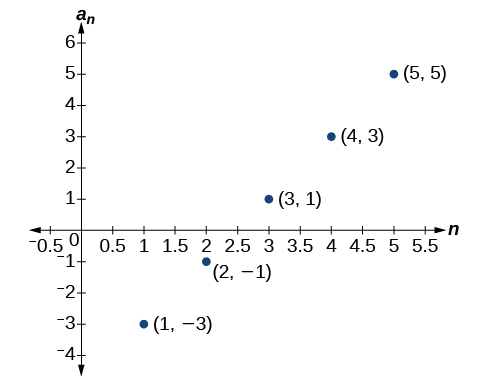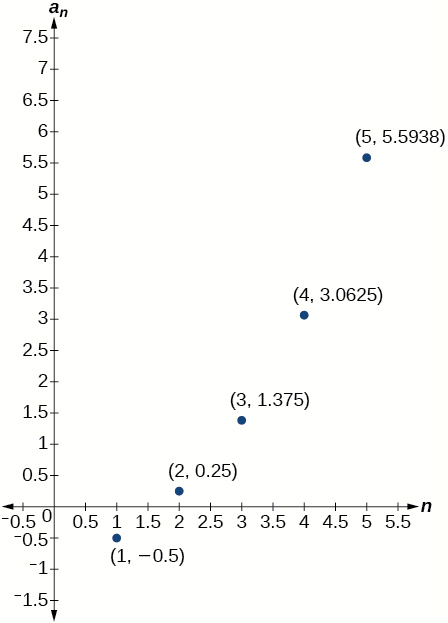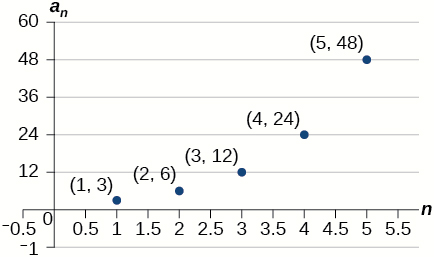# 13.3 Geometric sequences  (Page 4/6)

 Page 4 / 6

## Verbal

What is a geometric sequence?

A sequence in which the ratio between any two consecutive terms is constant.

How is the common ratio of a geometric sequence found?

What is the procedure for determining whether a sequence is geometric?

Divide each term in a sequence by the preceding term. If the resulting quotients are equal, then the sequence is geometric.

What is the difference between an arithmetic sequence and a geometric sequence?

Describe how exponential functions and geometric sequences are similar. How are they different?

Both geometric sequences and exponential functions have a constant ratio. However, their domains are not the same. Exponential functions are defined for all real numbers, and geometric sequences are defined only for positive integers. Another difference is that the base of a geometric sequence (the common ratio) can be negative, but the base of an exponential function must be positive.

## Algebraic

For the following exercises, find the common ratio for the geometric sequence.

$1,3,9,27,81,...$

$-0.125,0.25,-0.5,1,-2,...$

The common ratio is $-2$

$-2,-\frac{1}{2},-\frac{1}{8},-\frac{1}{32},-\frac{1}{128},...$

For the following exercises, determine whether the sequence is geometric. If so, find the common ratio.

$-6,-12,-24,-48,-96,...$

The sequence is geometric. The common ratio is 2.

$5,5.2,5.4,5.6,5.8,...$

$-1,\frac{1}{2},-\frac{1}{4},\frac{1}{8},-\frac{1}{16},...$

The sequence is geometric. The common ratio is $-\frac{1}{2}.$

$6,8,11,15,20,...$

$0.8,4,20,100,500,...$

The sequence is geometric. The common ratio is $5.$

For the following exercises, write the first five terms of the geometric sequence, given the first term and common ratio.

$\begin{array}{cc}{a}_{1}=8,& r=0.3\end{array}$

$\begin{array}{cc}{a}_{1}=5,& r=\frac{1}{5}\end{array}$

$5,1,\frac{1}{5},\frac{1}{25},\frac{1}{125}$

For the following exercises, write the first five terms of the geometric sequence, given any two terms.

$\begin{array}{cc}{a}_{7}=64,& {a}_{10}\end{array}=512$

$\begin{array}{cc}{a}_{6}=25,& {a}_{8}\end{array}=6.25$

$800,400,200,100,50$

For the following exercises, find the specified term for the geometric sequence, given the first term and common ratio.

The first term is $2,$ and the common ratio is $3.$ Find the 5 th term.

The first term is 16 and the common ratio is $-\frac{1}{3}.$ Find the 4 th term.

${a}_{4}=-\frac{16}{27}$

For the following exercises, find the specified term for the geometric sequence, given the first four terms.

${a}_{n}=\left\{-1,2,-4,8,...\right\}.$ Find ${a}_{12}.$

${a}_{n}=\left\{-2,\frac{2}{3},-\frac{2}{9},\frac{2}{27},...\right\}.$ Find ${a}_{7}.$

${a}_{7}=-\frac{2}{729}$

For the following exercises, write the first five terms of the geometric sequence.

$\begin{array}{cc}{a}_{1}=-486,& {a}_{n}=-\frac{1}{3}\end{array}{a}_{n-1}$

$\begin{array}{cc}{a}_{1}=7,& {a}_{n}=0.2{a}_{n-1}\end{array}$

$7,1.4,0.28,0.056,0.0112$

For the following exercises, write a recursive formula for each geometric sequence.

${a}_{n}=\left\{-1,5,-25,125,...\right\}$

${a}_{n}=\left\{-32,-16,-8,-4,...\right\}$

$\begin{array}{cc}a{}_{1}=-32,& {a}_{n}=\frac{1}{2}{a}_{n-1}\end{array}$

${a}_{n}=\left\{14,56,224,896,...\right\}$

${a}_{n}=\left\{10,-3,0.9,-0.27,...\right\}$

$\begin{array}{cc}{a}_{1}=10,& {a}_{n}=-0.3{a}_{n-1}\end{array}$

${a}_{n}=\left\{0.61,1.83,5.49,16.47,...\right\}$

${a}_{n}=\left\{\frac{3}{5},\frac{1}{10},\frac{1}{60},\frac{1}{360},...\right\}$

$\begin{array}{cc}{a}_{1}=\frac{3}{5},& {a}_{n}=\frac{1}{6}{a}_{n-1}\end{array}$

${a}_{n}=\left\{-2,\frac{4}{3},-\frac{8}{9},\frac{16}{27},...\right\}$

${a}_{n}=\left\{\frac{1}{512},-\frac{1}{128},\frac{1}{32},-\frac{1}{8},...\right\}$

${a}_{1}=\frac{1}{512},{a}_{n}=-4{a}_{n-1}$

For the following exercises, write the first five terms of the geometric sequence.

${a}_{n}=-4\cdot {5}^{n-1}$

${a}_{n}=12\cdot {\left(-\frac{1}{2}\right)}^{n-1}$

$12,-6,3,-\frac{3}{2},\frac{3}{4}$

For the following exercises, write an explicit formula for each geometric sequence.

${a}_{n}=\left\{-2,-4,-8,-16,...\right\}$

${a}_{n}=\left\{1,3,9,27,...\right\}$

${a}_{n}={3}^{n-1}$

${a}_{n}=\left\{-4,-12,-36,-108,...\right\}$

${a}_{n}=\left\{0.8,-4,20,-100,...\right\}$

${a}_{n}=0.8\cdot {\left(-5\right)}^{n-1}$

${a}_{n}=\left\{-1.25,-5,-20,-80,...\right\}$

${a}_{n}=\left\{-1,-\frac{4}{5},-\frac{16}{25},-\frac{64}{125},...\right\}$

${a}_{n}=-{\left(\frac{4}{5}\right)}^{n-1}$

${a}_{n}=\left\{2,\frac{1}{3},\frac{1}{18},\frac{1}{108},...\right\}$

${a}_{n}=\left\{3,-1,\frac{1}{3},-\frac{1}{9},...\right\}$

${a}_{n}=3\cdot {\left(-\frac{1}{3}\right)}^{n-1}$

For the following exercises, find the specified term for the geometric sequence given.

Let ${a}_{1}=4,$ ${a}_{n}=-3{a}_{n-1}.$ Find ${a}_{8}.$

Let ${a}_{n}=-{\left(-\frac{1}{3}\right)}^{n-1}.$ Find ${a}_{12}.$

${a}_{12}=\frac{1}{177,147}$

For the following exercises, find the number of terms in the given finite geometric sequence.

${a}_{n}=\left\{-1,3,-9,...,2187\right\}$

${a}_{n}=\left\{2,1,\frac{1}{2},...,\frac{1}{1024}\right\}$

There are $12$ terms in the sequence.

## Graphical

For the following exercises, determine whether the graph shown represents a geometric sequence.The graph does not represent a geometric sequence.

For the following exercises, use the information provided to graph the first five terms of the geometric sequence.

$\begin{array}{cc}{a}_{1}=1,& r=\frac{1}{2}\end{array}$

$\begin{array}{cc}{a}_{1}=3,& {a}_{n}=2{a}_{n-1}\end{array}$${a}_{n}=27\cdot {0.3}^{n-1}$

## Extensions

Use recursive formulas to give two examples of geometric sequences whose 3 rd terms are $\text{\hspace{0.17em}}200.$

Answers will vary. Examples: ${\begin{array}{cc}{a}_{1}=800,& {a}_{n}=0.5a\end{array}}_{n-1}$ and ${\begin{array}{cc}{a}_{1}=12.5,& {a}_{n}=4a\end{array}}_{n-1}$

Use explicit formulas to give two examples of geometric sequences whose 7 th terms are $1024.$

Find the 5 th term of the geometric sequence $\left\{b,4b,16b,...\right\}.$

${a}_{5}=256b$

Find the 7 th term of the geometric sequence $\left\{64a\left(-b\right),32a\left(-3b\right),16a\left(-9b\right),...\right\}.$

At which term does the sequence exceed $100?$

The sequence exceeds $100$ at the 14 th term, ${a}_{14}\approx 107.$

At which term does the sequence begin to have integer values?

For which term does the geometric sequence ${a}_{{}_{n}}=-36{\left(\frac{2}{3}\right)}^{n-1}$ first have a non-integer value?

${a}_{4}=-\frac{32}{3}\text{\hspace{0.17em}}$ is the first non-integer value

Use the recursive formula to write a geometric sequence whose common ratio is an integer. Show the first four terms, and then find the 10 th term.

Use the explicit formula to write a geometric sequence whose common ratio is a decimal number between 0 and 1. Show the first 4 terms, and then find the 8 th term.

Answers will vary. Example: Explicit formula with a decimal common ratio: ${a}_{n}=400\cdot {0.5}^{n-1};$ First 4 terms: $\begin{array}{cc}400,200,100,50;& {a}_{8}=3.125\end{array}$

Is it possible for a sequence to be both arithmetic and geometric? If so, give an example.

(1+cosA+IsinA)(1+cosB+isinB)/(cos@+isin@)(cos$+isin$)
hatdog
Mark
how we can draw three triangles of distinctly different shapes. All the angles will be cutt off each triangle and placed side by side with vertices touching
bsc F. y algebra and trigonometry pepper 2
given that x= 3/5 find sin 3x
4
DB
remove any signs and collect terms of -2(8a-3b-c)
-16a+6b+2c
Will
Joeval
(x2-2x+8)-4(x2-3x+5)
sorry
Miranda
x²-2x+9-4x²+12x-20 -3x²+10x+11
Miranda
x²-2x+9-4x²+12x-20 -3x²+10x+11
Miranda
(X2-2X+8)-4(X2-3X+5)=0 ?
master
The anwser is imaginary number if you want to know The anwser of the expression you must arrange The expression and use quadratic formula To find the answer
master
The anwser is imaginary number if you want to know The anwser of the expression you must arrange The expression and use quadratic formula To find the answer
master
Y
master
master
Soo sorry (5±Root11* i)/3
master
Mukhtar
2x²-6x+1=0
Ife
explain and give four example of hyperbolic function
What is the correct rational algebraic expression of the given "a fraction whose denominator is 10 more than the numerator y?
y/y+10
Mr
Find nth derivative of eax sin (bx + c).
Find area common to the parabola y2 = 4ax and x2 = 4ay.
Anurag
y2=4ax= y=4ax/2. y=2ax
akash
A rectangular garden is 25ft wide. if its area is 1125ft, what is the length of the garden
to find the length I divide the area by the wide wich means 1125ft/25ft=45
Miranda
thanks
Jhovie
What do you call a relation where each element in the domain is related to only one value in the range by some rules?
A banana.
Yaona
given 4cot thither +3=0and 0°<thither <180° use a sketch to determine the value of the following a)cos thither
what are you up to?
nothing up todat yet
Miranda
hi
jai
hello
jai
Miranda Drice
jai
aap konsi country se ho
jai
which language is that
Miranda
I am living in india
jai
good
Miranda
what is the formula for calculating algebraic
I think the formula for calculating algebraic is the statement of the equality of two expression stimulate by a set of addition, multiplication, soustraction, division, raising to a power and extraction of Root. U believe by having those in the equation you will be in measure to calculate it
Miranda
state and prove Cayley hamilton therom
hello
Propessor
hi
Miranda
the Cayley hamilton Theorem state if A is a square matrix and if f(x) is its characterics polynomial then f(x)=0 in another ways evey square matrix is a root of its chatacteristics polynomial.
Miranda
hi
jai
hi Miranda
jai
thanks
Propessor
welcome
jai

#### Get Jobilize Job Search Mobile App in your pocket Now!ByByBy Rohini AjayBy Vanessa SoledadBy OpenStaxBy Mahee BooBy OpenStaxBy Anonymous UserBy Jonathan LongBy RhodesBy Danielle StephensBy Candice Butts